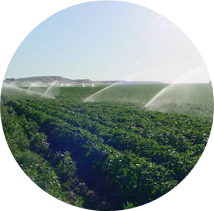# Sprinkler Calculators

Sprinkler Application Rate: Sprinkler application rate based on nozzle diameter and spacing.

Nozzle Requirements: Find the flow rate using a given pressure and diameter, or find the nozzle diameter for a given pressure and flow rate.

Lateral Friction Loss: Find the flow rate, pipe length and friction loss based on a sprinkler lateral.

Hand Move Sprinklers: Find recommended values for hand move sprinklers, such as set times, nozzle and pressure losses.

Sprinkler Density: Find the number of sprinkler heads per area for rectangular or triangular spacing.

Sprinkler Discharge Rate: Learn how to estimate a single sprinkler's discharge rate by setting up an experiment using two sprinklers, 4 cans and a measuring container.

Traveling Big Gun Sprinkler: Calculate the net water application for a given flow rate, efficiency percentage, lane width, and travel speed.

Traveling Sprinkler: Estimate the the total number of irrigated acres per hour based on lane spacing and travel speed.WSU Prosser - IAREC, 24106 N Bunn Rd, Prosser WA 99350-8694, 509-786-2226,